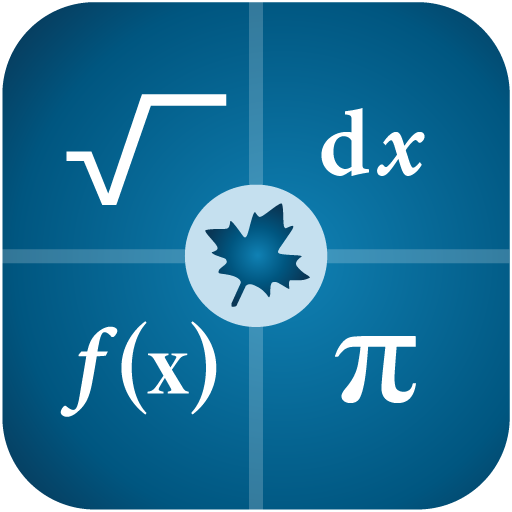# Maple Calculator: Math Solver

##### About Maple Calculator: Math Solver
Powered by Maple, the most powerful math engine in the world, this all-in-one calculator solves math problems, generates 2-D and 3-D visualizations, and provides step-by-step solutions for a wide variety of math homework problems encountered at high school and university.

💯STEP BY STEP MATH SOLUTIONS FOR HOMEWORK] This app is a graphing calculator, scientific calculator, algebra calculator, calculus calculator, and integration calculator combined all into one! Take a picture of your problem using your phone's camera or enter it via the app's built-in math editor to see the final answer or get step-by-step solutions.

[⚡️QUICK AND POWERFUL MATH SOLVER] No matter how you enter your problem, you can find derivatives and integrals, factor polynomials, invert matrices, solve systems of equations, solve ODEs, and much more. Our calculator has the power of the world-leading Maple math engine behind it, so it can do a LOT of math!

[📊GRAPH PROBLEMS AND RESULTS] See 2-D and 3-D graphs of your expressions instantly, and watch how the graph changes as you change the expression. On this calculator you can zoom in, pan, and even rotate 3-D plots to get a closer look at areas of interest.

[🧩PLAY A BUILT-IN FUN MATH GAME]Play our calculator’s built in game, Sumzle, which is like Wordle but for math and equations.

FEATURES:
• Enter Math Problems Using Your Camera Or By Drawing them with the Handwriting Palette Or By Entering Directly with the Built-in Math Keyboard
• Do All Sorts Of Math Operations And Get Step By Step Solutions
• Get Answers Even When You Are Offline
• Take Quality Math Notes Through Maple Learn. Use The Calculator Camera To Automatically Send Your Handwritten Steps To Maple Learn Where You Can Uncover Mistakes And Share Your Work With Others.
• You Can Upload Mathematical Expressions From Our Calculator To Maple Desktop
• International Language Support (English, Spanish, French, German, Russian, Danish, Swedish, Japanese, Hindi, And Simplified Chinese)

MATH CAPABILITIES ON OUR CALCULATOR:
• Basic Math: Arithmetic, Fractions, Decimals, Integers, Factors, Square Roots, Powers
• Algebra: Solving And Graphing Linear Equations, Solving And Graphing Systems Of Equations, Working With Polynomials, Quadratic Equations, And Functions, Logarithmic And Exponential Functions, Trigonometric Functions, Trigonometric Identities
• Precalculus: Graphing, Piecewise Functions, Absolute Value, Inequalities, Implicit Functions
• Linear Algebra: Finding The Determinant, Inverse, Transpose, Eigenvalues, And Eigenvectors, Solving Matrices (Reduced Echelon Form & Gaussian Elimination)
• Differential Equations: Solving Ordinary Differential Equations
• And more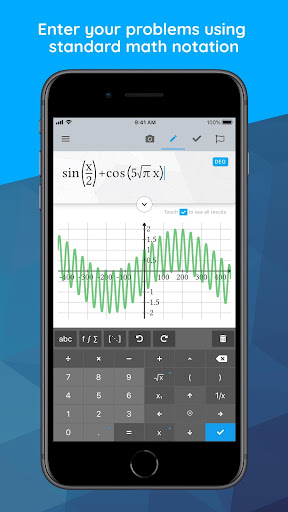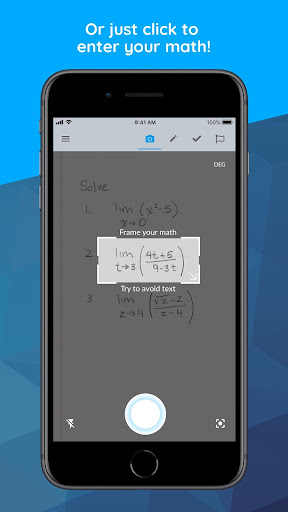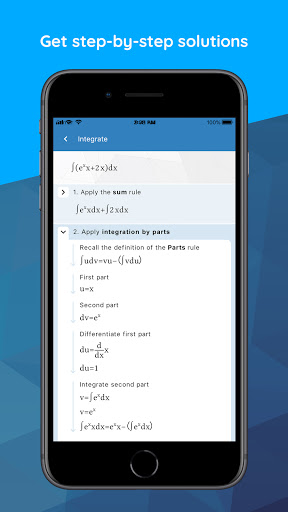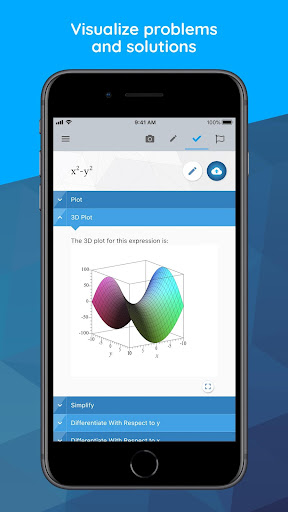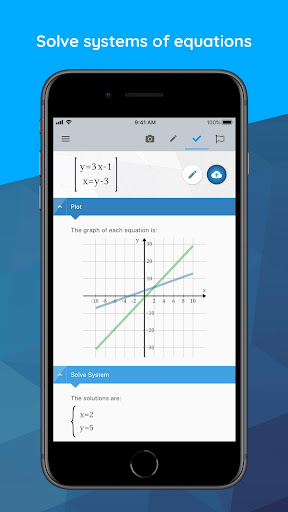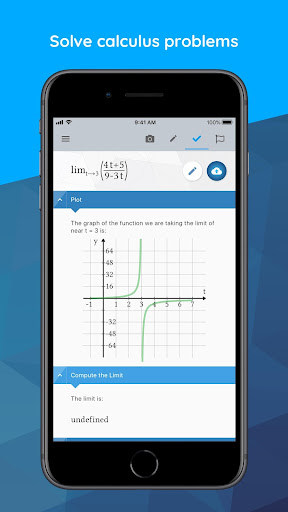##### Recent Updates - Version 3.3.3
• Try the new handwriting recognition tool..
Fixed a bug which stopped plots from appearing on the results screen.